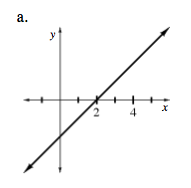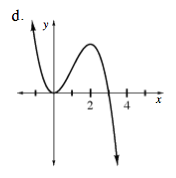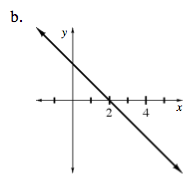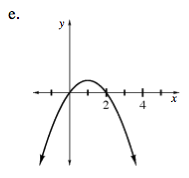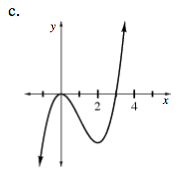### Home > CALC > Chapter 8 > Lesson 8.2.3 > Problem8-92

8-92.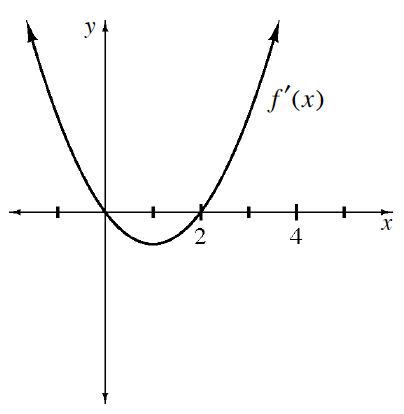Multiple Choice: The graph of f ′(x) is shown at right. Which of the graphs below could be the graph of f(x) ? Homework Help ✎

 a.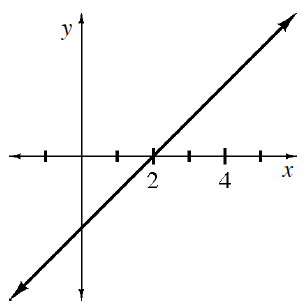b.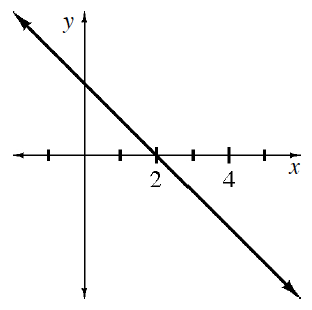c.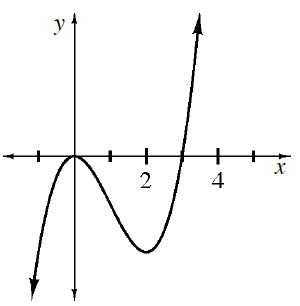d.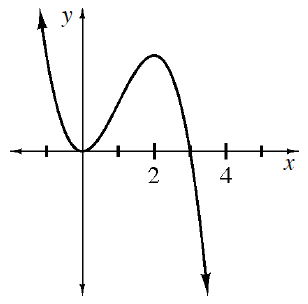e.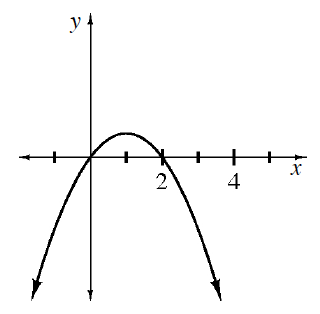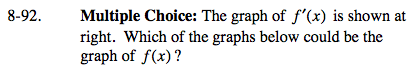f '(x) appears to be a parabola. What family of functions has a derivative that is a parabola?

f '(x) shows the slopes of f(x). What is significant about the slopes before x = 0 and after x = 2?